# ICSE Class 8 Maths Selina Solutions for Chapter 19 Representing 3D in 2D

In our day to day life, we come across various objects with different shapes and sizes like triangles, squares, circles, etc. Some of these shapes which have length, breadth and height are called 3D or three-dimensional objects. Other objects like a sheet of paper which have length and breadth are called two-dimensional or 2D shapes. Chapter 19 Representing 3D in 2D explains about how three-dimensional objects can be represented into 2-dimensional objects along with suitable examples related to the concept.

The ICSE Class 8 Maths Selina Solutions Chapter 19 Representing 3D in 2D are very helpful for students from the exam point of view. Here we have provided the solutions of all the questions mentioned in this chapter prepared by our subject experts. These Selina Solutions are solved in a step by step manner so that students can refer to these solutions while solving the textbook questions.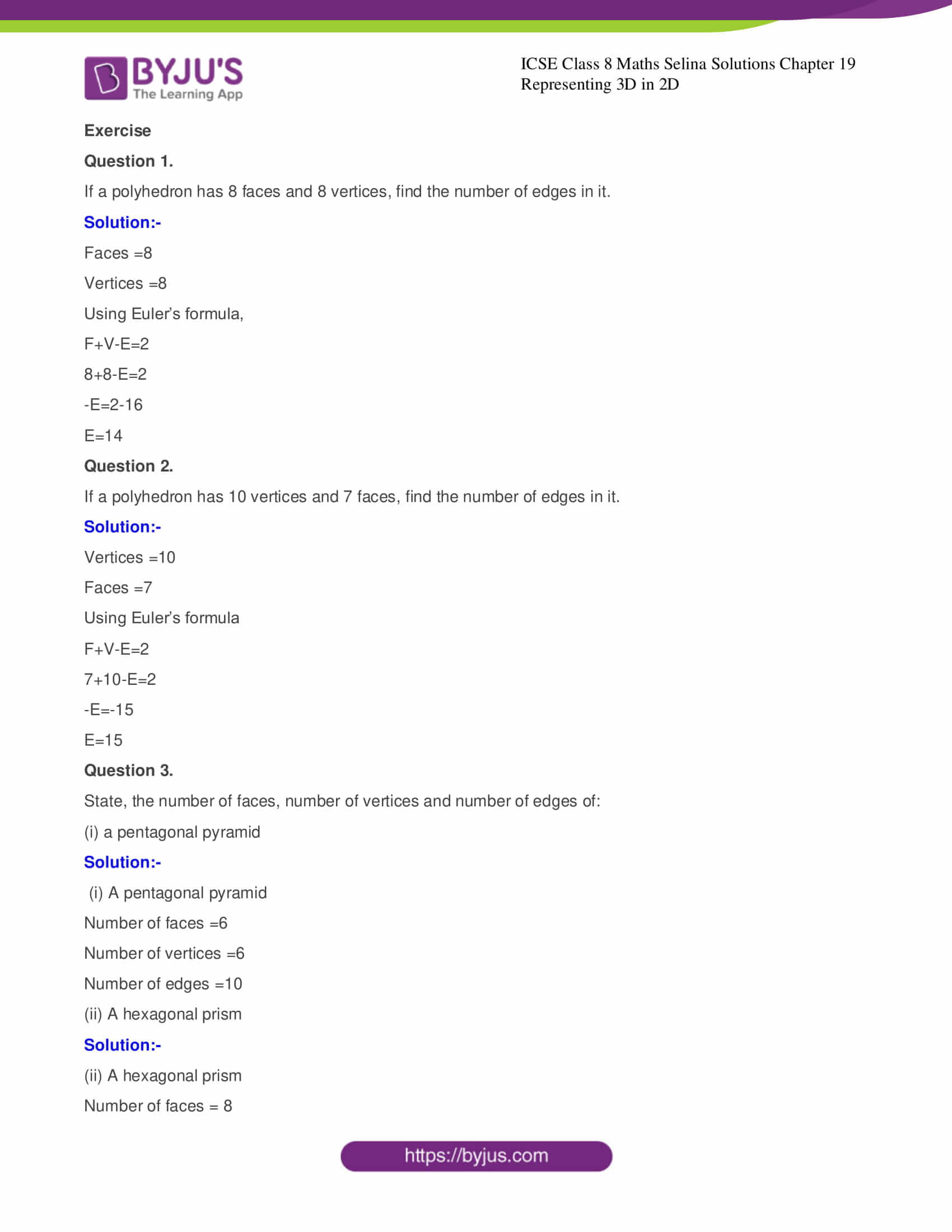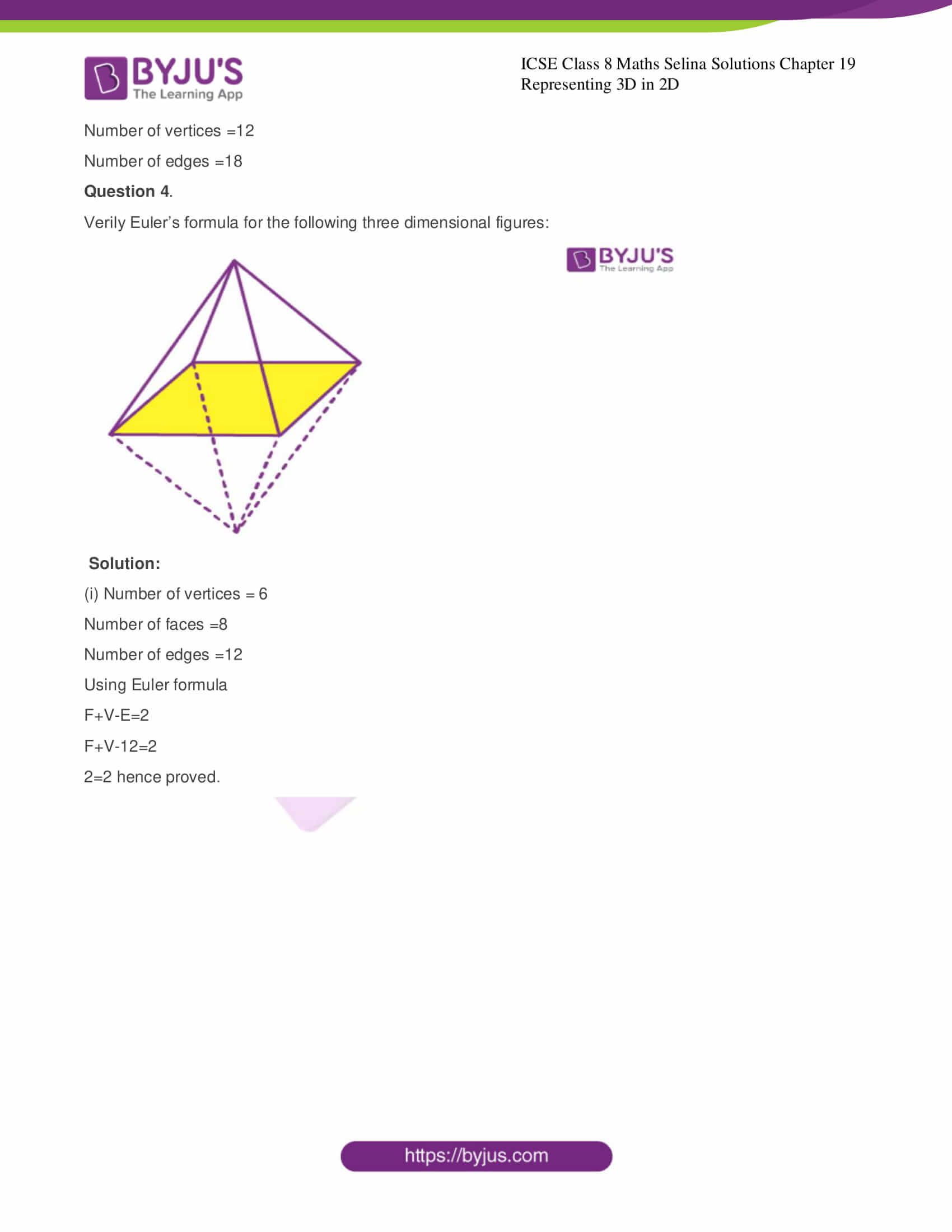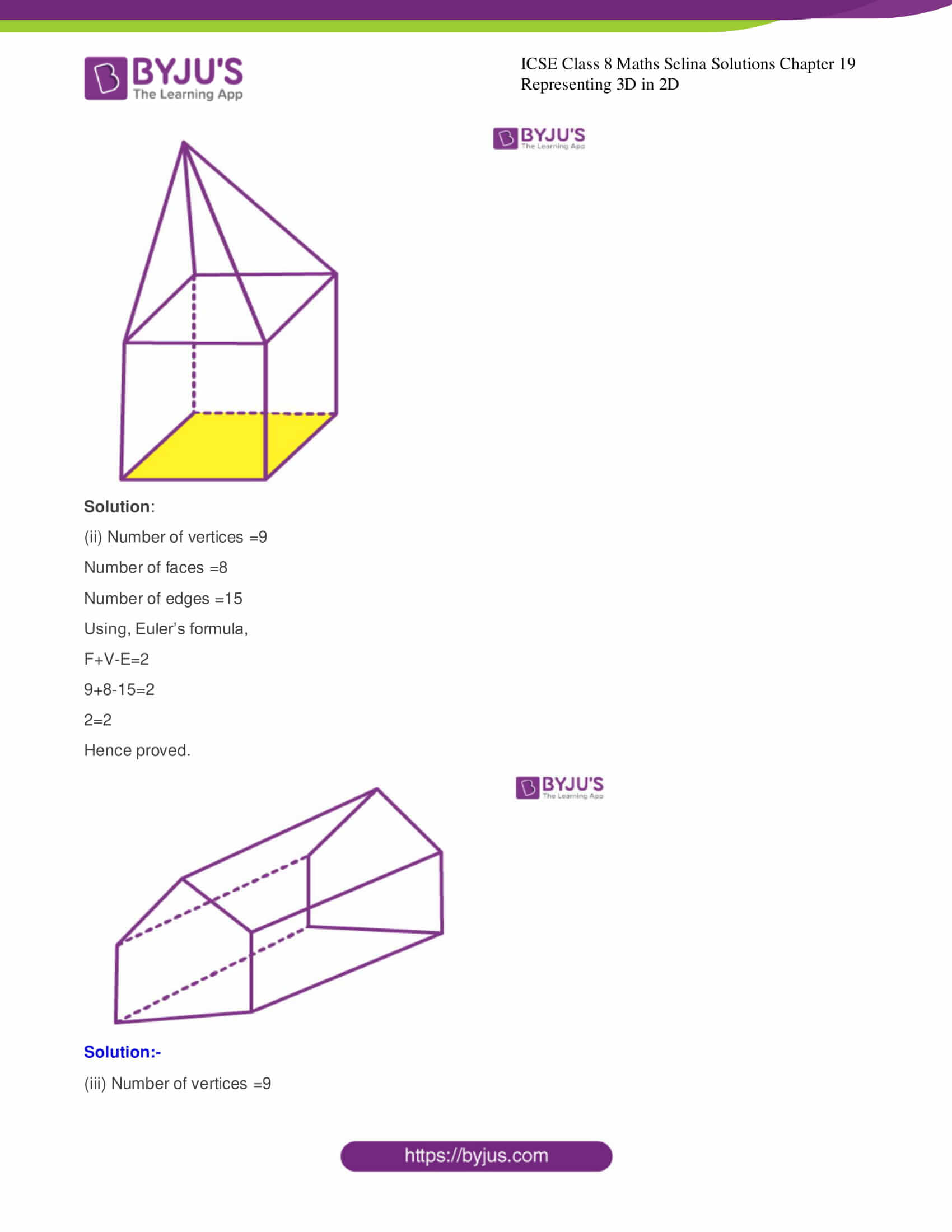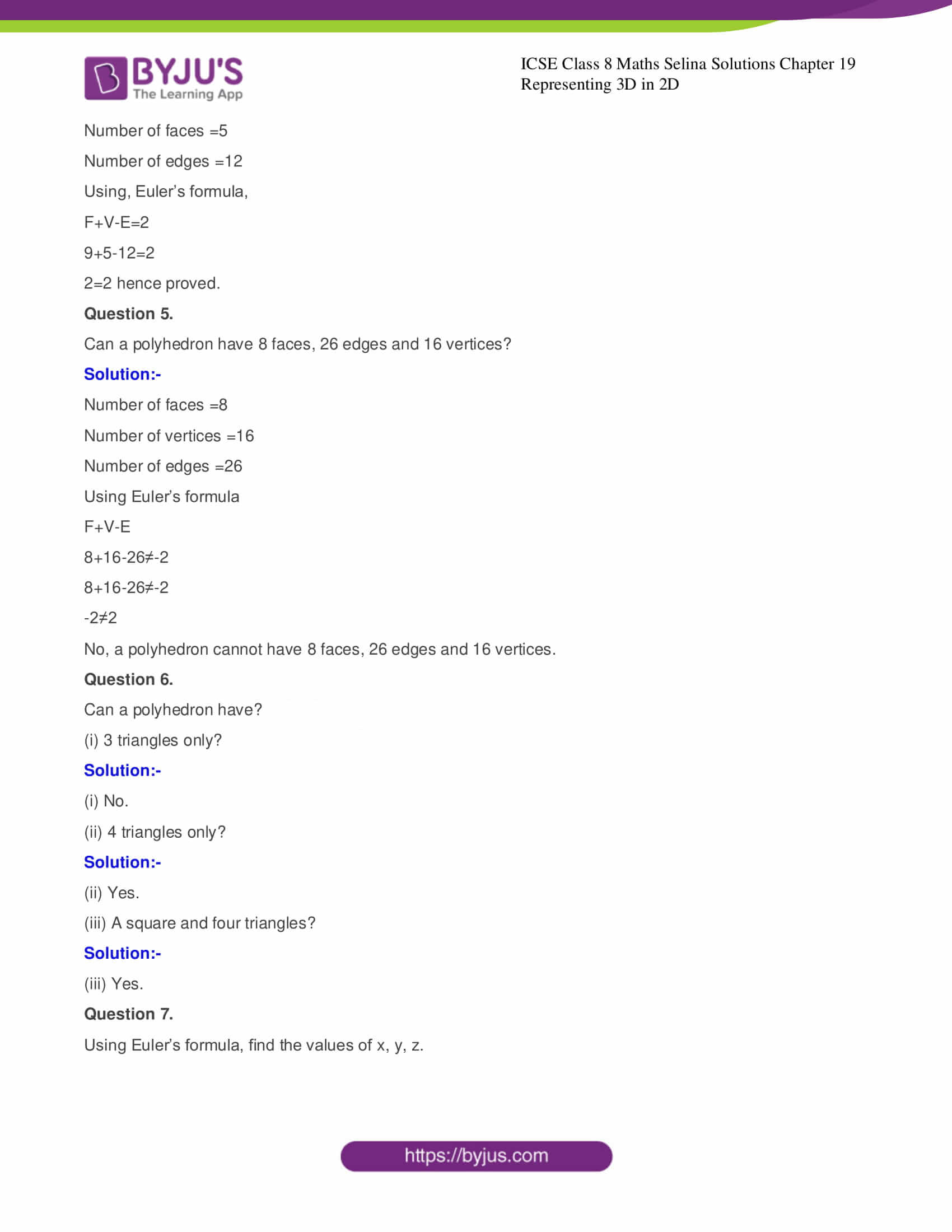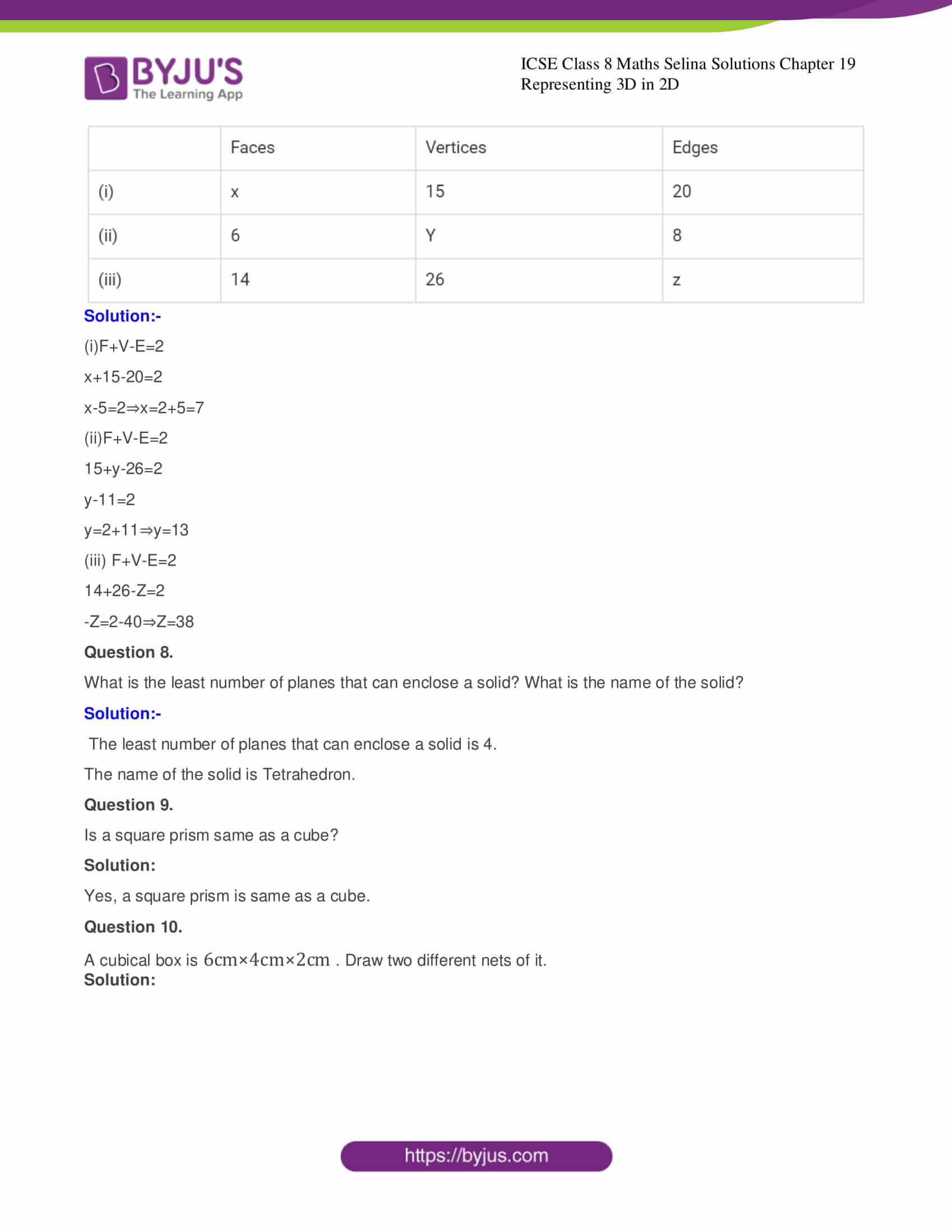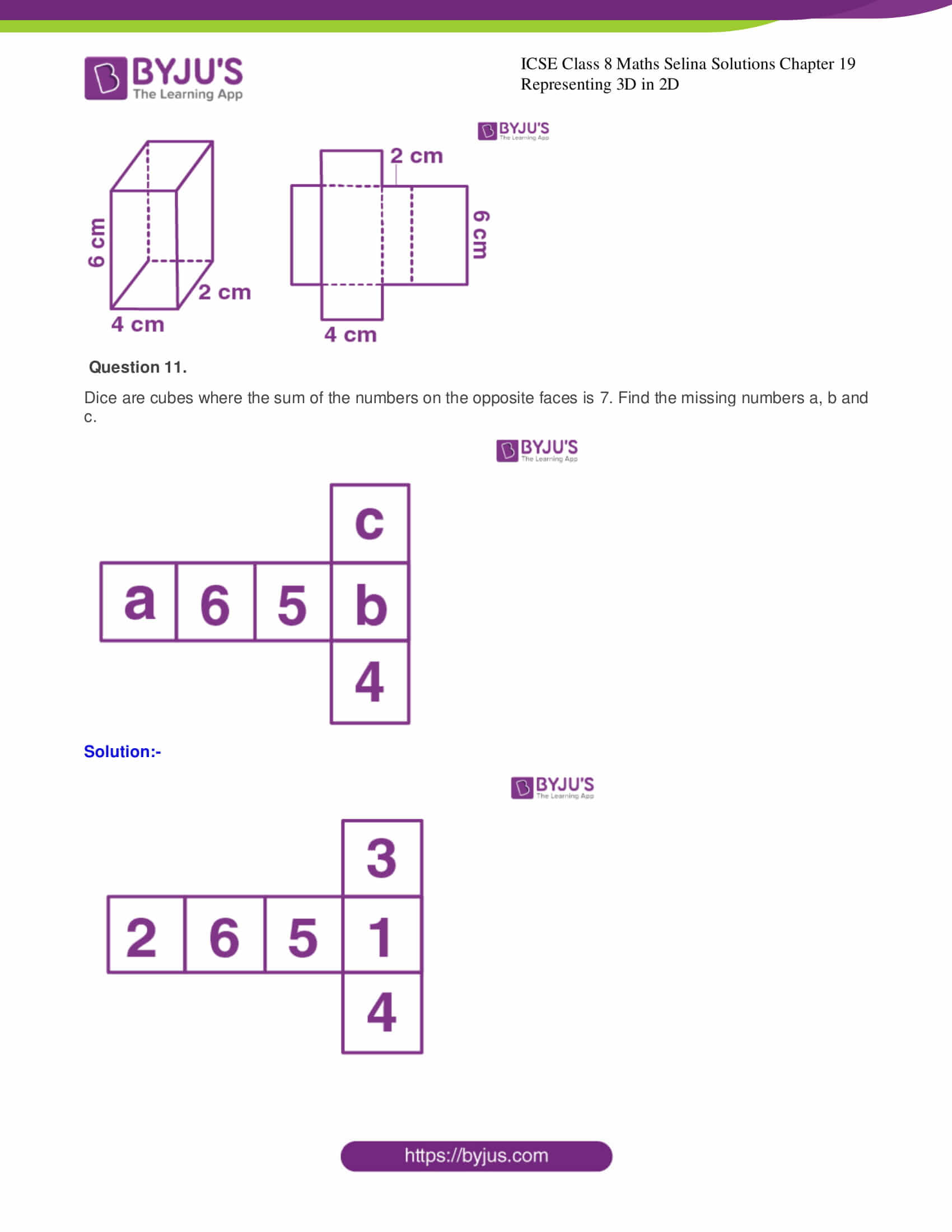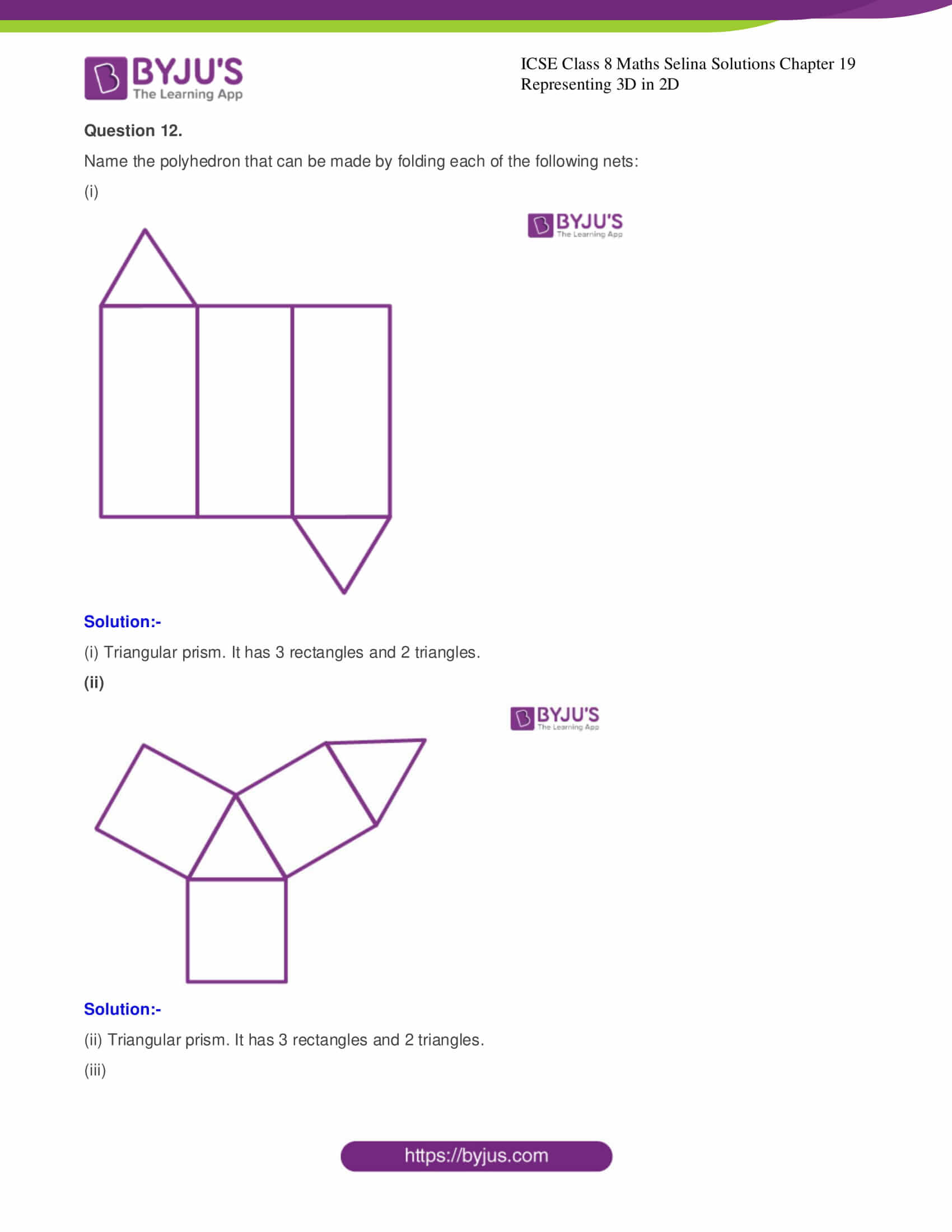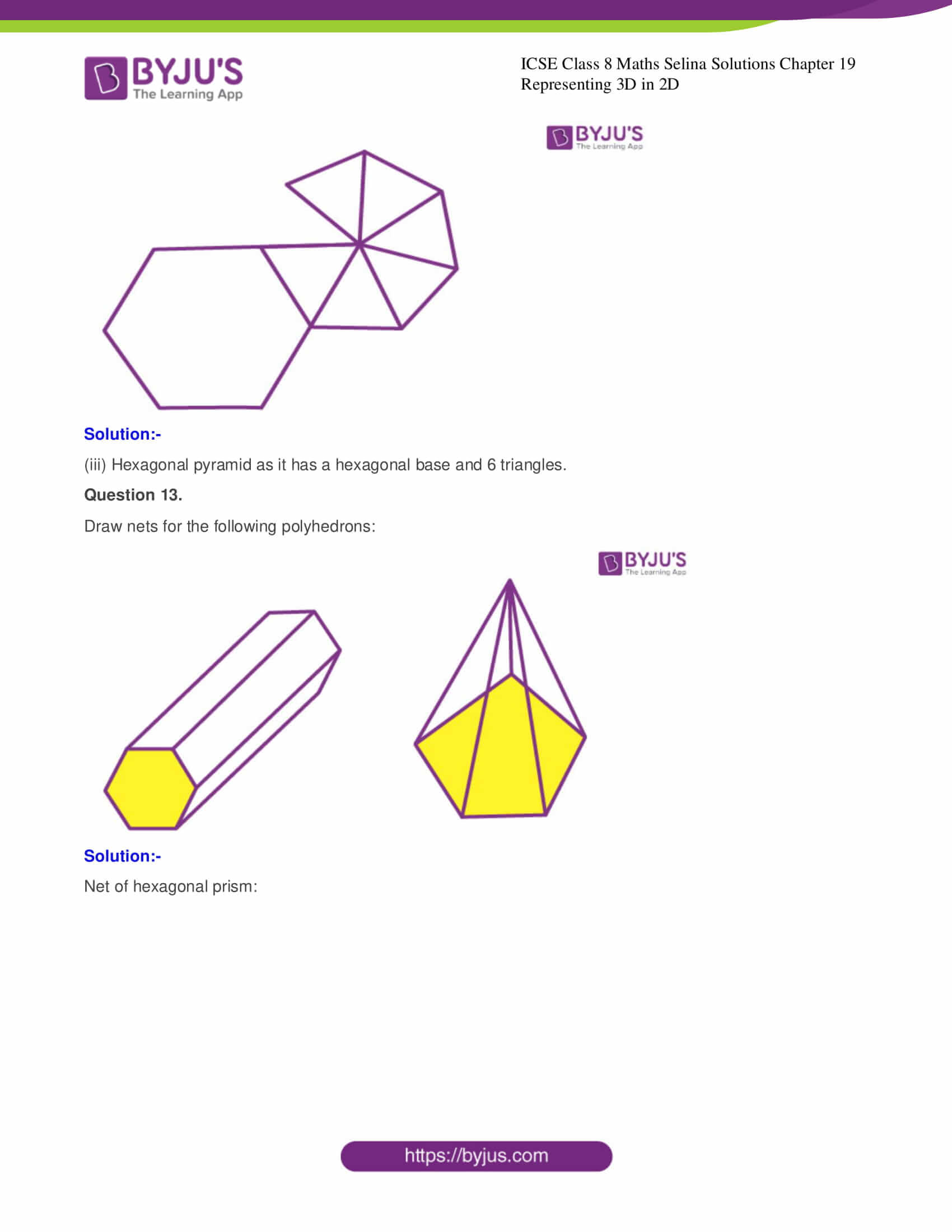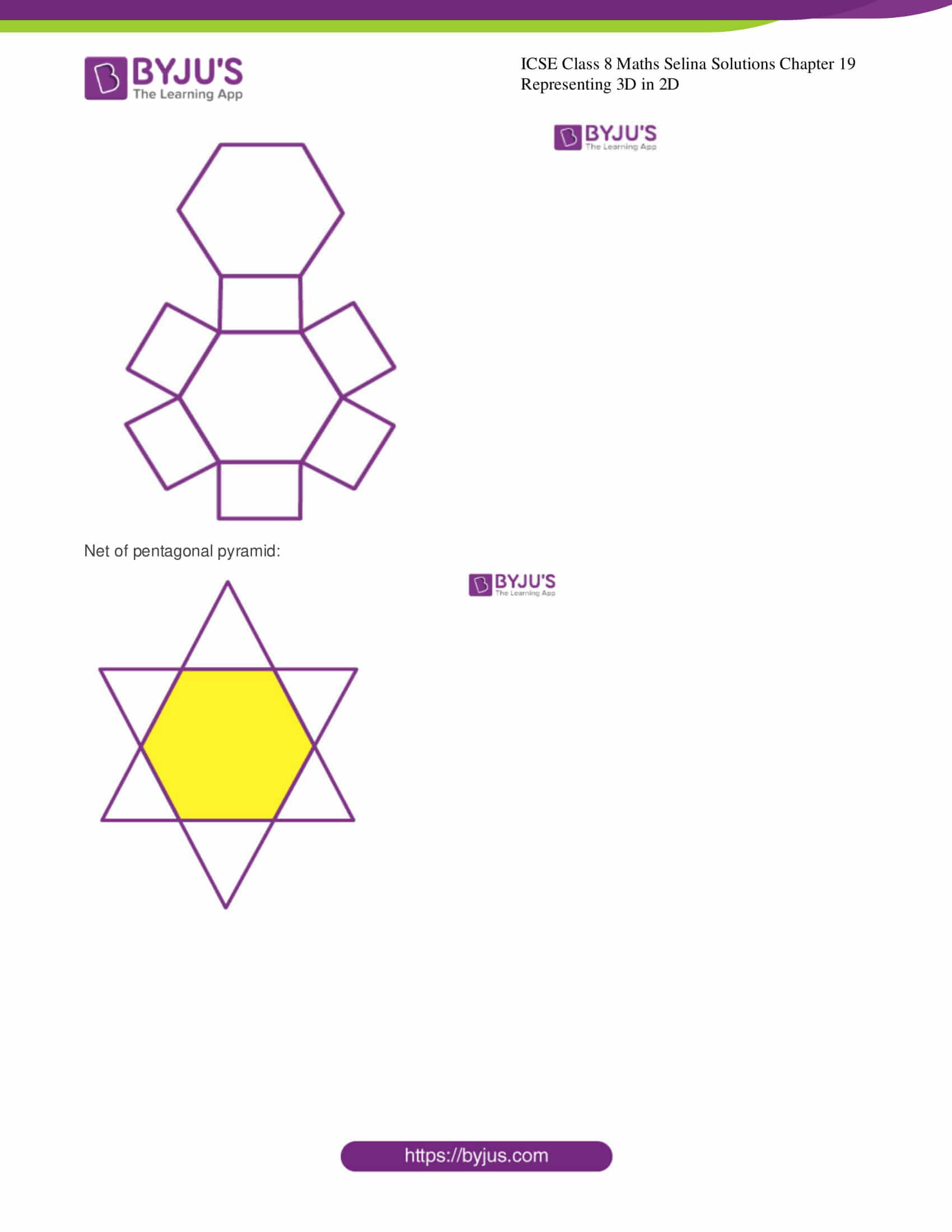ICSE Class 8 Maths Selina Solutions Chapter 19 Representing 3D in 2D consists of 15 questions and detailed solution of all questions are provided below.

### CHAPTER 19 – REPRESENTING 3-D IN 2-D

Exercise

Question 1.

If a polyhedron has 8 faces and 8 vertices, find the number of edges in it.

Solution:-

Faces =8

Vertices =8

Using Euler’s formula,

F+V-E=2

8+8-E=2

-E=2-16

E=14

Question 2.

If a polyhedron has 10 vertices and 7 faces, find the number of edges in it.

Solution:-

Vertices =10

Faces =7

Using Euler’s formula

F+V-E=2

7+10-E=2

-E=-15

E=15

Question 3.

State, the number of faces, number of vertices and number of edges of:

(i) a pentagonal pyramid

Solution:-

(i) A pentagonal pyramid

Number of faces =6

Number of vertices =6

Number of edges =10

(ii) A hexagonal prism

Solution:-

(ii) A hexagonal prism

Number of faces = 8

Number of vertices =12

Number of edges =18

Question 4.

Verily Euler’s formula for the following three dimensional figures: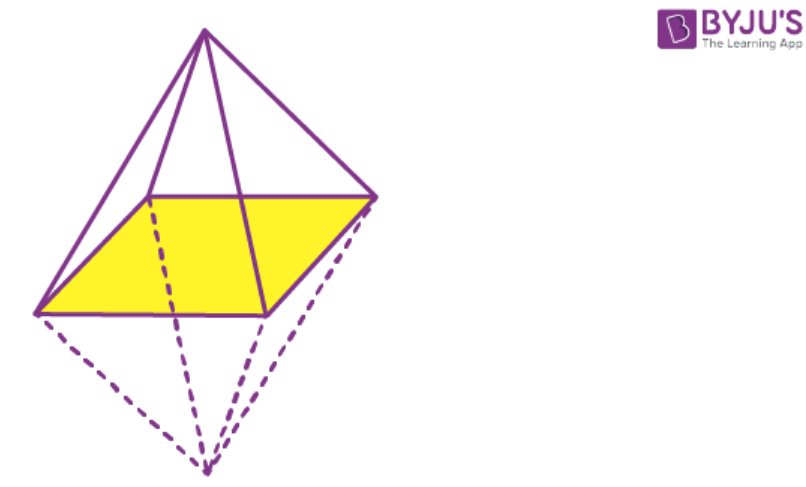Solution:

(i) Number of vertices = 6

Number of faces =8

Number of edges =12

Using Euler formula

F+V-E=2

F+V-12=2

2=2 hence proved.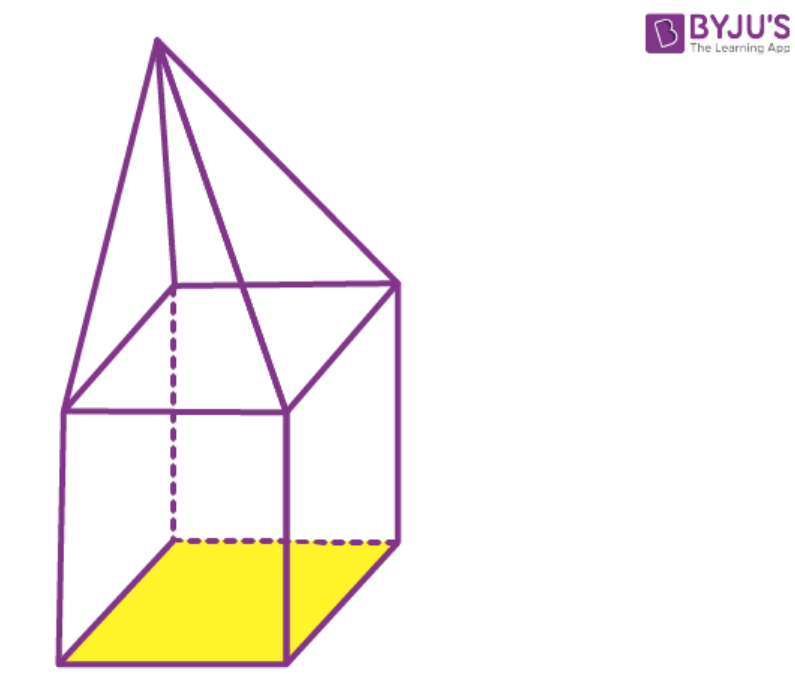Solution:

(ii) Number of vertices =9

Number of faces =8

Number of edges =15

Using, Euler’s formula,

F+V-E=2

9+8-15=2

2=2

Hence proved.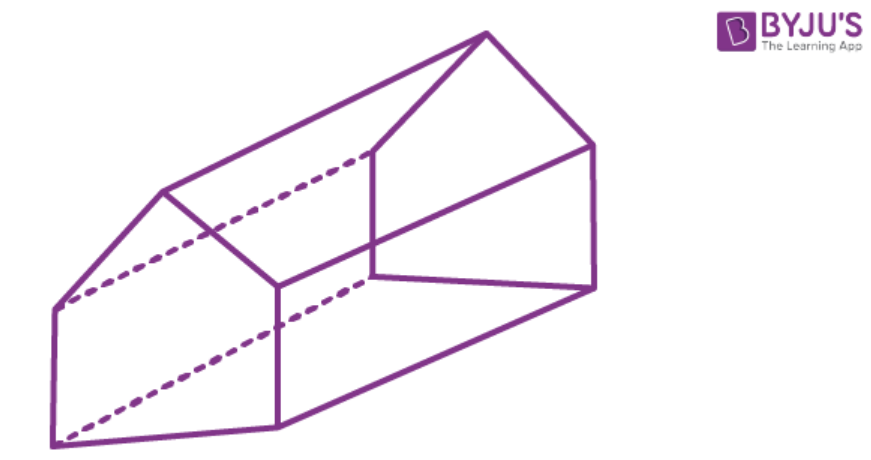Solution:-

(iii) Number of vertices =9

Number of faces =5

Number of edges =12

Using, Euler’s formula,

F+V-E=2

9+5-12=2

2=2 hence proved.

Question 5.

Can a polyhedron have 8 faces, 26 edges and 16 vertices?

Solution:-

Number of faces =8

Number of vertices =16

Number of edges =26

Using Euler’s formula

F+V-E

8+16-26≠-2

8+16-26≠-2

-2≠2

No, a polyhedron cannot have 8 faces, 26 edges and 16 vertices.

Question 6.

Can a polyhedron have?

(i) 3 triangles only?

Solution:-

(i) No.

(ii) 4 triangles only?

Solution:-

(ii) Yes.

(iii) A square and four triangles?

Solution:-

(iii) Yes.

Question 7.

Using Euler’s formula, find the values of x, y, z.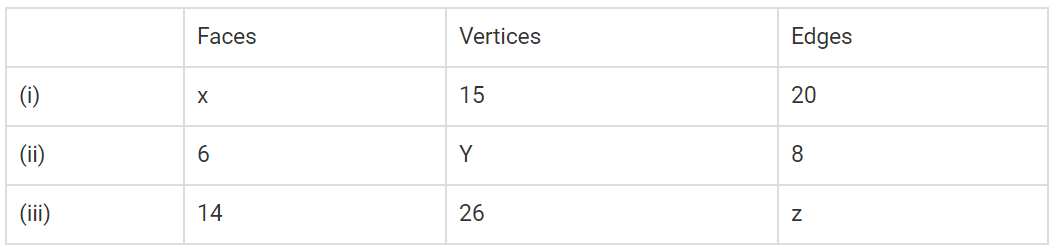Solution:-

(i)F+V-E=2

x+15-20=2

x-5=2⇒x=2+5=7

(ii)F+V-E=2

15+y-26=2

y-11=2

y=2+11⇒y=13

(iii) F+V-E=2

14+26-Z=2

-Z=2-40⇒Z=38

Question 8.

What is the least number of planes that can enclose a solid? What is the name of the solid?

Solution:-

The least number of planes that can enclose a solid is 4.

The name of the solid is Tetrahedron.

Question 9.

Is a square prism same as a cube?

Solution:

Yes, a square prism is same as a cube.

Question 10.

A cubical box is 6cm×4cm×2cm . Draw two different nets of it.

Solution: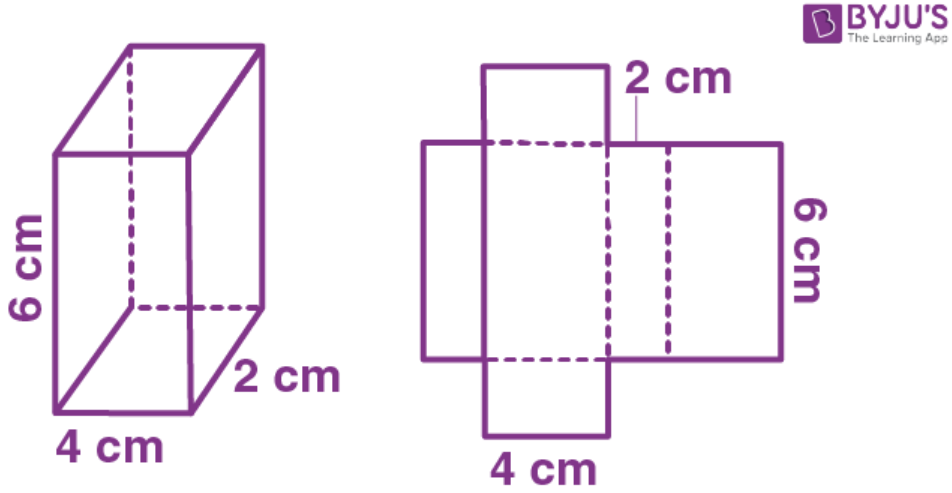Question 11.

Dice are cubes where the sum of the numbers on the opposite faces is 7. Find the missing numbers a, b and c.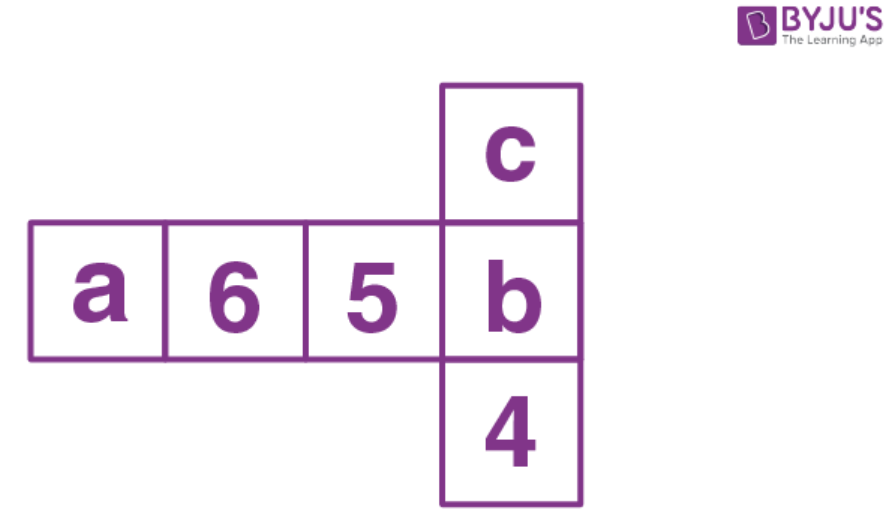Solution:-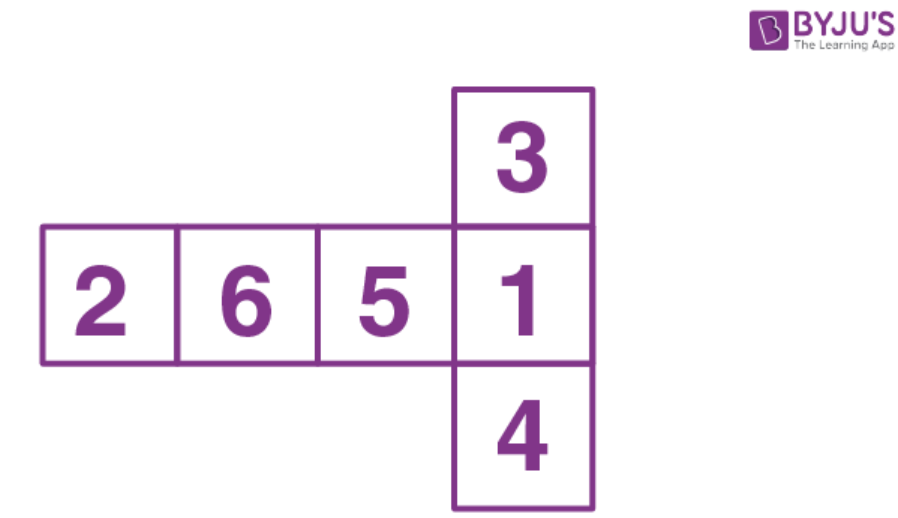Question 12.

Name the polyhedron that can be made by folding each of the following nets:

(i)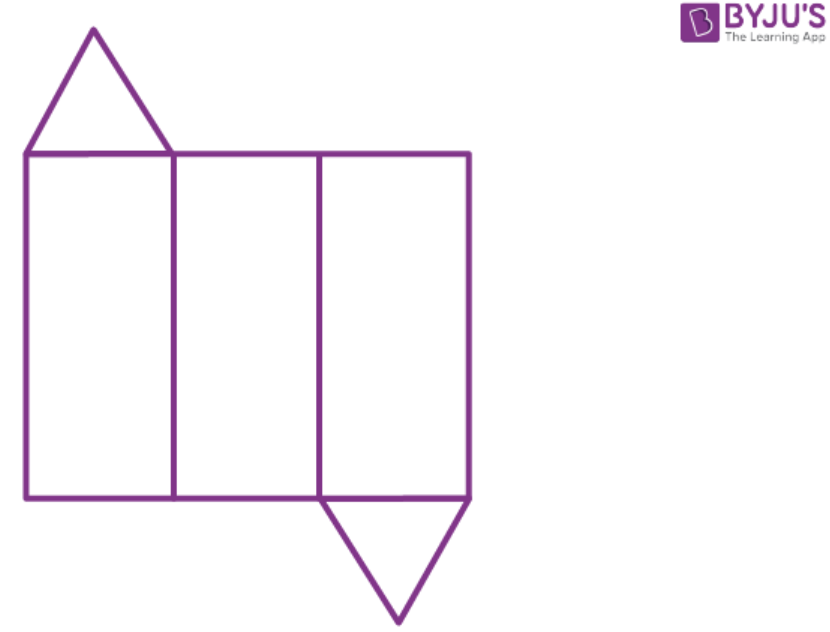Solution:-

(i) Triangular prism. It has 3 rectangles and 2 triangles.

(ii)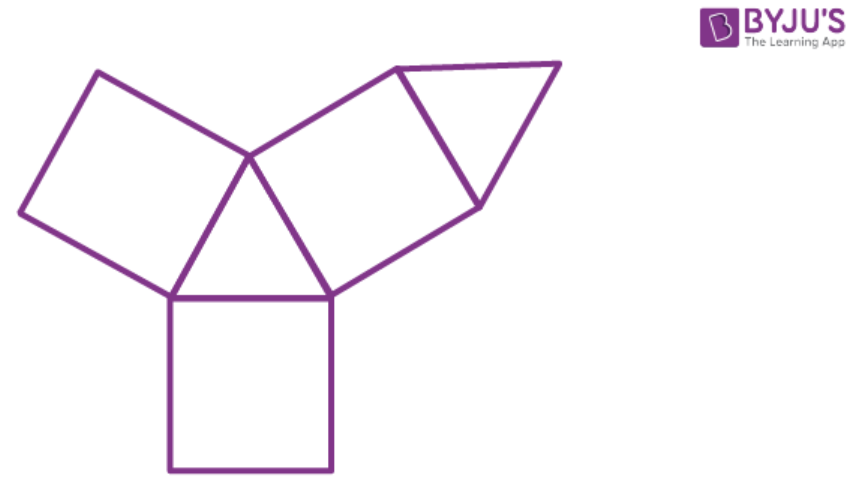Solution:-

(ii) Triangular prism. It has 3 rectangles and 2 triangles.

(iii)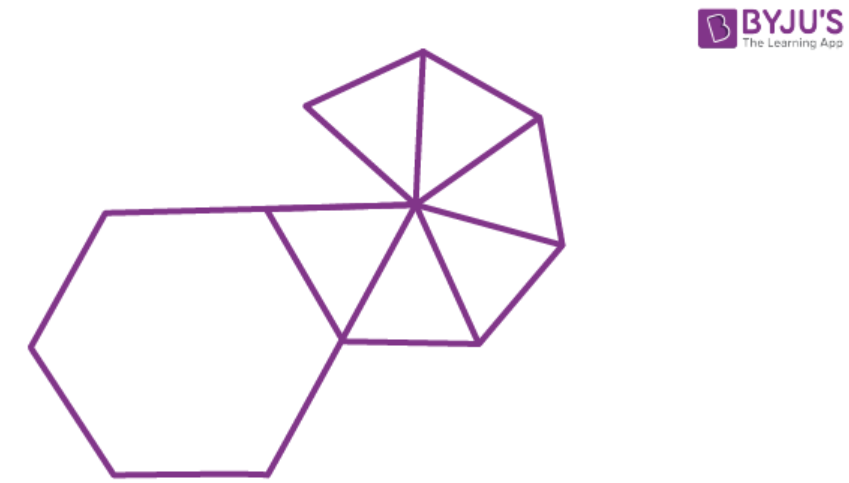Solution:-

(iii) Hexagonal pyramid as it has a hexagonal base and 6 triangles.

Question 13.

Draw nets for the following polyhedrons: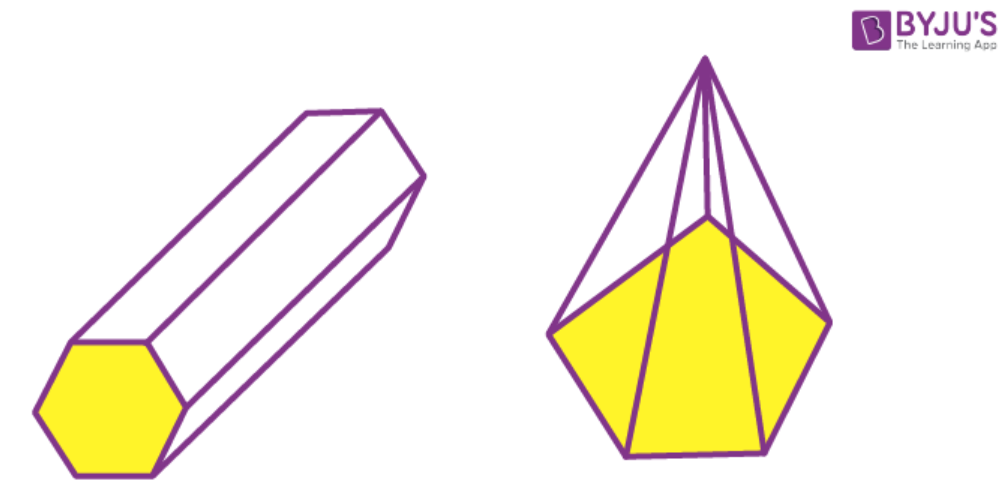Solution:-

Net of hexagonal prism: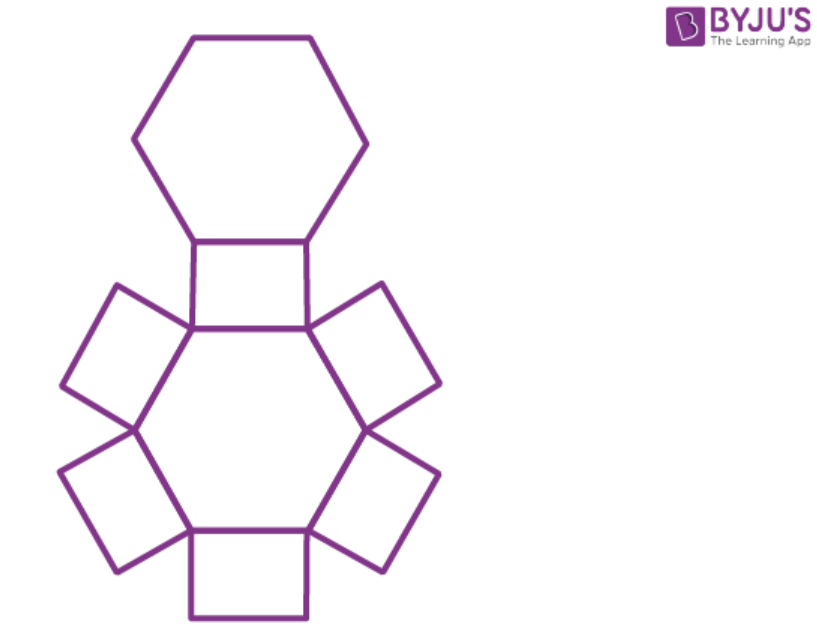Net of pentagonal pyramid: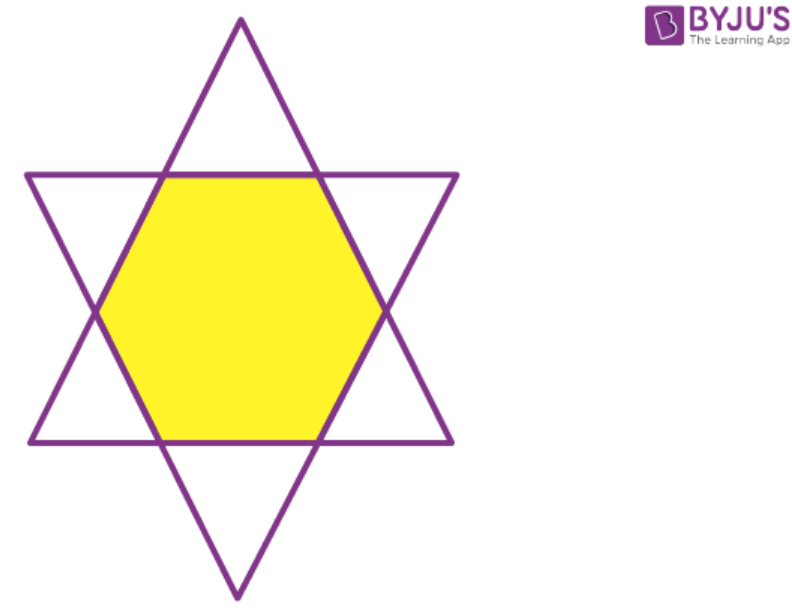### ICSE Class 8 Maths Selina Solutions Chapter 19 – Representing 3D in 2D

In chapter 19 Representing 3D in 2D students will learn Euler’s formula, two-dimensional shapes, three-dimensional shapes, view of 3D shapes, etc. The concepts covered in the chapter gives a basic introduction so that students have some knowledge about it before they step into the next class. The solutions define each and every topic in a simple and understandable manner so that students don’t get confused while going through the chapter.

To access solutions of Physics, Chemistry and Biology subjects of class 8 click on ICSE Class 8 Selina Solutions. Keep learning and stay tuned for further updates on ICSE and other competitive exams. To access interactive Maths and Science Videos download BYJU’S App and subscribe to YouTube Channel.